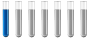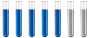## Calculating dilution ratio of sulfuric acid and water

Chemistry and homework help forum.

Organic Chemistry, Analytical Chemistry, Biochemistry, Physical Chemistry, Computational Chemistry, Theoretical Chemistry, High School Chemistry, Colledge Chemistry and University Chemistry Forum.

Share your chemistry ideas, discuss chemical problems, ask for help with scientific chemistry questions, inspire others by your chemistry vision!

Please feel free to start a scientific chemistry discussion here!

Discuss chemistry homework problems with experts!

Ask for help with chemical questions and help others with your chemistry knowledge!

Moderators: Xen, expert, ChenBeier

coryk
NewbiePosts: 4
Joined: Tue Jun 03, 2008 10:47 am

### Calculating dilution ratio of sulfuric acid and water

Hi

This is my first time posting. I am trying to figure a problem that was giving to me at work, but I'm not a chemistry major and I did some searching through my chemistry book, but could not find anything to start with. Here is my delima, I have sulfuric acid at 149 Fahrenheit mix with water at ambient temperature, what ratio of water to sulfuric acid do I need to maintain the solution (acid & water) to 120 Fahrenheit? Any idea would be very helpful and appreciated as I am delaying my project for this.

I should note that the project is to figure out which material to use for draining out the solution, PVDF or Teflon fittings.
Zedekiah
Staff MemberPosts: 77
Joined: Thu May 01, 2008 5:33 pm
If I understand correctly, you need to know how much (room temperature) water to add to a mixture at 149 F to reduce the temperature to 120 F.

If this is not the case, you're going to need to be more specific.

To solve this problem conclusively, we would need to know the concentration of sulfuric acid in the mixture. This is important because adding water to concentrated sulfuric acid will cause the the temperature to rise significantly.

Now. We know that the heat of a solution is related to :

q = mC(dT)

We don't know 'C' in this case if we don't know the concentration of the original mixture, unfortunately.

Assuming it is close to water :

(100)(4.186)(-16.12) = q
q = (x)(4.186)(27.77)

(100)(-16.12)=x(27.77)

x = 58 grams of room temperature water per 100 grams of reaction mixture to lower the temperature from 149 F to 120 F.

But this is a really rough approximation, I need a little more information about the situation to finalize the solution!
coryk
NewbiePosts: 4
Joined: Tue Jun 03, 2008 10:47 am
Yes, I need to know how much (room temperature) water to add to sulfuric acid @ 98% concentration from 149 F to reduce the temperature to 120 F (mixture of sulfuric acid + water). I beleive from looking up the data table, the specific heat C=1.465 J/g*C. for 98% concentration.

Does this calcultions include the heat produced from the exothermic reaction? I'm curious where did the figures (-16.12) and (27.77) in the equation come from? Isn't the delta T for water (ambient temp of 75 - 120 ) = -45, and the delta T for sulfuric acid is (149 - 120) = 29?
coryk
NewbiePosts: 4
Joined: Tue Jun 03, 2008 10:47 amI did not the convert the temperature to celcius. Pardon my confusion. Thanks so much for the help. Greatly appreciate it.
Zedekiah
Staff MemberPosts: 77
Joined: Thu May 01, 2008 5:33 pm
This value does not include the heat of solution. I will be able to solve this problem momentarily, that is plenty of data.
Zedekiah
Staff MemberPosts: 77
Joined: Thu May 01, 2008 5:33 pm
H2SO4 => HSO4(-) + H(+)

From the CRC Handbook of Chemistry and Physics, the dH of this reaction is, according to Hess' Law, -95.34 kJ / mol.

Since we can assume any value of moles as long as we keep it consistent, let's do one mole of sulfuric acid and convert this to milliliters later.

-95,340 J is the deficit to begin with. (Oh yeah, forgot to mention, I will be using Celsius and convert back to imperial units at the end).

Initial acid temperature : 65 C
Initial water temperature : 23.8... C
Final temperatures : 48.8... C

q = mCdT

One expression involves the sulfuric acid and the other involves the water.

A mole of sulfuric acid is, coincidentally, approximately 100 grams in a 98% solution. (The molar mass of sulfuric acid is 98.078 g / mol and 98% of 100 grams of solution is 98 grams.)

Sulfuric acid :

(100 g)(1.465 J/g*C)(-16.2 C) = q

Water :
(X g)(4.186 J/g*C)(-25 C) = q

(100 g)(1.465 J/g*C)(-16.2 C) = (X g)(4.186 J/g*C)(-25 C)

-2,373.3 J = (X g)(4.186 J/g*C)(-25 C)

Now add in the heat of solution that we need to get rid of :

-95,340 J + -2,373.3 J = (X g)(4.186 J/g*C)(-25 C)

-97,713 J = (X g)(4.186 J/g*C)(-25 C)

993.7 grams of water per 100.0 grams of 98% sulfuric acid.

Keep in mind the heat of solution of sulfuric acid accounts for 971 grams by itself.
Last edited by Zedekiah on Tue Jun 03, 2008 7:44 pm, edited 1 time in total.
Zedekiah
Staff MemberPosts: 77
Joined: Thu May 01, 2008 5:33 pm
Note : This means 55 ml of sulfuric acid for 1 liter of water.

Another note : the sulfuric acid, after this dilution, is going to be approximately 9% concentrated.

Final note : The error of this calculation is likely on the side of caution, this amount of water assumes complete dissociation of 98% sulfuric acid, which may already have a fraction dissociated because of its 2% water content.
coryk
NewbiePosts: 4
Joined: Tue Jun 03, 2008 10:47 am
well done Mr. Zedekiah,

I attempted to work this out at home after work. After remembering your comment of including the heat of solution or total heat of enthalphy, I couldn't figure out what to do with the dH, then it just come to my senses that that heat shuold be added to the system or the equation.

You have guide me to the light. Thanks again, I'm glad of getting something out of this project.
emmausa
NewbiePosts: 3
Joined: Tue Oct 17, 2023 7:36 pm

### Re: Calculating dilution ratio of sulfuric acid and water

good
ChenBeier
Distinguished MemberPosts: 1420
Joined: Wed Sep 27, 2017 7:25 am
Location: Berlin, Germany